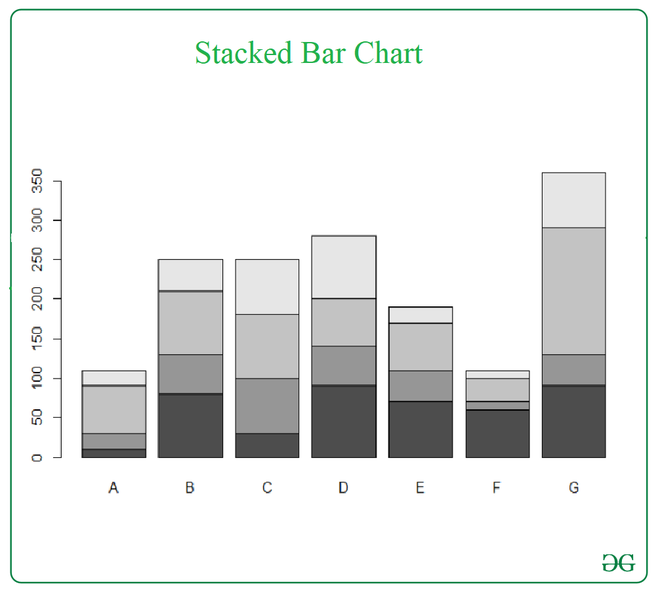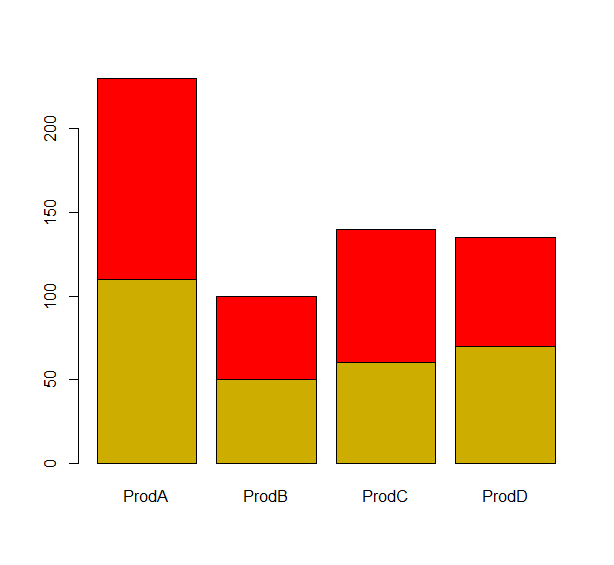Open in App
Not now

# Stacked Bar Chart in R

• Last Updated : 17 Feb, 2021

A stacked bar chart extends the standard bar chart from looking at numeric values across one categorical variable to two. Each bar in a standard bar chart is divided into a number of sub-bars stacked end to end, each one corresponding to a level of the second categorical variable. This article discusses how one can be created using R.

The function used here to create a stacked bar chart is barplot().

Syntax: barplot(H,xlab,ylab,main, names.arg,col)

Parameters:

• H: is a vector or matrix containing numeric values used in a bar chart.
• xlab: is the label for the x-axis.
• ylab: is the label for the y-axis.
• main: is the title of the bar chart.
• names.arg: is a vector of names appearing under each bar.
• col: is used to give colors to the bars in the graph.

Returns: a barplot.

Approach

• Create data
• Pass the data to the barplot() function
• Pass appropriate arguments to the function
• Display plot

Example:

## R

 `dat <- ``read.table``(text = "A   B   C   D   E   F    G``1 10 80 30 90 70 60  90``2 20 50 70 50 40 10  40``3 60 80 80 60  60 30 160``4 20 40 70 80 20 10  70", header = ``TRUE``)`` ` `barplot``(``as.matrix``(dat))`

Output:Example 2:

## R

 `dat <- ``read.table``(text ="ProdA ProdB ProdC ProdD``1 110 50 60 70 ``2 120 50 80 65", header= ``TRUE``)`` ` `barplot``(``as.matrix``(dat),col=``c``(``"gold3"``,``"red"``))`

Output:My Personal Notes arrow_drop_up